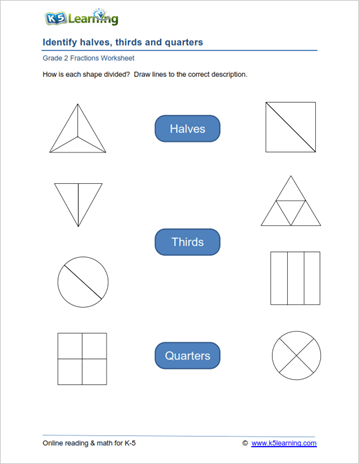# 2nd Grade Math Worksheets: Fractions

Our grade 2 fraction worksheets introduce students to fractions as both parts of a whole and parts of a set.  We cover identifying common fractions, comparing common fractions, and reading / writing fractions.

## Equal parts of a whole

Equal parts worksheets (halves, thirds, quarters ....)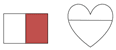Numerator and denominator of a fraction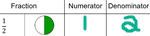Writing fractions from a numerator and denominator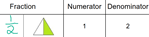Reading fractions and matching to words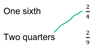Writing fractions in words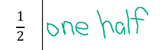## Identifying common fractions

Choose the fraction that matches the picture

Choose the picture that matches the fraction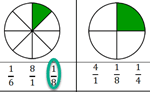## Parts of a set

Coloring parts of a group to match a fraction

Writing fractions to represent parts of a set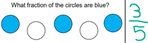Match fractions to a set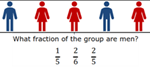## Comparing fractions using pictures

Compare fractions (parts of a whole, same denominator)

Compare fractions (same numerators)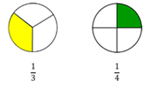Comparing fractions using block diagrams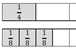Comparing fractions (parts of a set)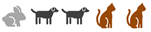## Fraction word problems

Basic fraction word problems
Writing and comparing fractions from a story